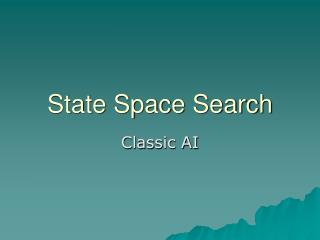DownloadDownload PresentationState Space Search

# State Space Search

Télécharger la présentation## State Space Search

- - - - - - - - - - - - - - - - - - - - - - - - - - - E N D - - - - - - - - - - - - - - - - - - - - - - - - - - -
##### Presentation Transcript

1. State Space Search Classic AI

2. State Space representation of a problem is a graph • Nodes correspond to problem states • Arcs correspond to steps in a solution process • One node corresponds to an initial state • One node corresponds to a goal state

3. Solution Path An ordered sequence of nodes from the initial state to the goal state

4. Search Algorithm Finds a solution path through a state space

5. The Water Jug Problem Suppose we have • An empty 4 gallon jug • An empty 3 gallon jug • A source of water • A task: put 2 gallons of water in the 4 gallon jug

6. Representation • State Space • Node on the graph is an ordered pair (x,y) • X is the contents of the 4 gallon jug • Y is the contents of the 3 gallon jug • Intitial State: (0,0) • Goal State: (2,N) N ε {0, 1, 2, 3}

7. Rules • if x < 4, fill x : (x,y)  (4,y) • if y < 3, fill y : (x,y)  (x,3) • if x > 0, empty x : (x,y)  (0,y) • if y > 0, empty y : (x,y)  (x,0) • if (x+y) >= 4 and y > 0 fill the 4 gallon jug from the 3 gallon jug (x,y)  (4, y – (4 – x)) • if (x+y) >= 3 and x > 0 Fill the 3 gallon jug from the 4 gallon jug (x,y)  (x –(3 – y), 3)) • if (x+y) <= 4 and y > 0 Pour the 3 gallon jug into the 4 gallon jug: (x,y)  (x+y), 0) • if (x+y) <= 3 and x > 0 pour the 4 gallon jug into the 3 gallon jug: (x,y)  (0, x + y)

8. Is there a solution path? Initial State: (0,0) Goal State: (2,N)

9. Breadth First Search (0,3) 1 2 (0,3) (4,0) (0,3) 6 7 2 (3,0) (4,3) (1,3) etc

10. Depth First (0,0) (4,0) 1 2 3 (4,3) 7 (0,3) (3,0) 2 (3,3) Etc. and without visiting already visited states

11. Backward/Forward Chaining Search can proceed • From data to goal • From goal to data Either could result in a successful search path, but one or the other might require examining more nodes depending on the circumstances

12. Data to goal is called forward chaining for data driven search Goal to data is called backward chaining or goal driven search

13. Examples • Water jug was data driven • Grandfather problem was goal driven • To make water jug goal driven: • Begin at (2,y) • Determine how many rules could produce this goal • Follow these rules backwards to the start state

14. Object • Reduce the size of the search space

15. Use Goal Driven • if • Goal is clearly stated • Many rules match the given facts • For example: the number of rules that conlude a given theorem is much smaller than the number that may be applied to the entire axiom set

16. Use Data Driven • If • Most data is given at the outset • Only a few ways to use the facts • Difficult to form an initial hypothesis • For example: DENDRAL, an expert system that finds molecular structure of organic compounds based on spectrographic data. There are lots of final possibilities, but only a few ways to use the initial data • Said another way: initial data constrains search